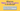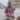# What are Higher-Order Functions in JavaScript?

Posted October 7, 2021Start discussion

The usual way you think about JavaScript functions is as reusable pieces of code that make some calculations. The arguments are the function’s input data, and the return value is the output.

Here’s a simple function that sums an array of numbers:

`js`function calculate(numbers) {  let sum = 0;  for (const number of numbers) {    sum = sum + number;  }  return sum;}calculate([1, 2, 4]); // => 7``

The numbers are the input, and the function `calculate()` returns the sum — the output.

What about implementing a more universal function that would support more operations on numbers: addition, multiplication, and more. How would you implement that?

Let’s see what the concept of the higher-order functions is, and how it can make `calculate()` function more universal in regards to operations it can support.

## 1. Higher-order functions

Let’s make a pause and think a bit about fundamentals.

In JavaScript, the functions can use primitive types (like numbers, strings), objects (like arrays, plain objects, regular expressions, etc) as arguments, and return a primitive type or object too.

In the previous example, `calculate([1, 2, 4])` accepts an array of numbers as an argument, and returns the number `7` — the sum.

But is it possible to use functions as values? Assign functions themselves to variables, use them as arguments, or even return? Yes, that’s possible!

All because functions in JavaScript are first-class citizens. This means that you can:

A. Assign functions to variables:

`javascript`// Assign to variablesconst hiFunction = function() {   return 'Hello!' };hiFunction(); // => 'Hello!'``

B. Use functions as arguments to other functions:

`javascript`// Use as argumentsfunction iUseFunction(func) {  return func();}iUseFunction(function () { return 42 }); // => 42``

C. And even return functions from functions:

`javascript`// Return function from functionfunction iReturnFunction() {  return function() { return 42 };}const myFunc = iReturnFunction();myFunc(); // => 42``

Finally, here’s the interesting part:

The functions that use other functions as arguments or return functions are named higher-order functions.

In the previous examples, `iUseFunction()` is higher-order because it accepts a function as an argument. Also `iReturnFunction()` is a higher-order function because it returns another function.

On the other side, the functions that use only primitives or objects as arguments, and only return primitives or objects are named first-order functions.

In the previous examples, `hiFunction()` is a first-order function since it simply returns a number.

So, in JavaScript a function can be either first-order or high-order.

That’s interesting, but why are higher-order functions useful? Let’s find out next!

## 2. The higher-order functions in practice

Let’s recall the question from the post introduction. How to make the `calculate()` function support multiple operations on the array of numbers?

The answer is to make `calculate()` a higher-order function, and supply dynamically the operation in form of a function as an argument.

Let’s modify the function to make it happen:

`javascript`function calculate(operation, initialValue, numbers) {  let total = initialValue;  for (const number of numbers) {    total = operation(total, number);  }  return total;}function sum(n1, n2) {  return n1 + n2;}function multiply(n1, n2) {  return n1 * n2;}calculate(sum, 0, [1, 2, 4]);      // => 7calculate(multiply, 1, [1, 2, 4]); // => 8``

Try the demo.

Here’s what changed: `calculate(operation, initialValue, numbers)` accepts the first argument a function that describes the operation, the second argument as the initial value, and finally the third argument is the array of numbers.

Now `calculate(operation, initialValue, numbers)` is a higher-order function because it accepts a function as the first argument.

`sum()` is the function that describes the addition operation. `calculate(sum, 0, [1, 2, 4])` is using this function to perform the sum of numbers.

Same way `multiply()` describes the multiplication operation. `calculate(multiply, 1, [1, 2, 4])` is using `multiply()` function to perform the production of all numbers.

### 2.1 The benefits of higher-order functions

What’s great is you can reuse the `calculate()` function to support multiple operations by providing different operation functions: addition, multiplication, and more.

Additionally, the concept of the higher-order function allows composability of functions. For example, you compose `calculate()` with `sum()` to calculate the sum of all numbers in an array. If you want to calculate the production, then you compose `calculate()` and `multiply()`.

In conclusion, higher-order functions enable better reusability and composability of functions. As result, they reduce the code duplication and favor the single-responsibility principle.

### 2.2 Higher-order functions and callbacks

Note that in the invocation `calculate(sum, 0, [1, 2, 4])`, the function `sum` supplied as an argument is also called a callback function.

In other words, you could think that a higher-order function accepts or returns callback functions.

## 3. Examples of higher-order functions

If you look closer at the built-in JavaScript function on arrays, strings, DOM methods, promise method — you could notice that many of them are higher-order functions as soon as they accept a function as an argument.

For example, the `array.map(mapperFunc)` method is a higher-order function because it accepts a mapper function as an argument:

`javascript`const numbers = [1, 2, 4];const doubles = numbers.map(function mapper(number) {  return 2 * number;});doubles; // [2, 4, 8]``

`element.addEventListener(type, handler)` DOM method is also a higher-order function since it accepts as the second argument the event handler function:

`javascript`document  .getElementById('#myButton')  .addEventListener('click', function handler() {    console.log('The button was clicked!');  });``

## 4. Conclusion

Higher-order functions in JavaScript are a special category of functions that either accept functions as an argument or return functions.

On the other side, if the function uses only primitives or objects as arguments or return values, these functions are first-order.

Higher-order functions provide the reusability benefit: the main behavior is provided by the higher-order function itself, and by accepting a function as an argument you extend that behavior at your will.

Challenge: Is there a built-in higher-order method on the array object similar to `calculate(operation, initialValue, numbers)`? Write your guess in a comment below!

## Quality posts into your inbox

I regularly publish posts containing:

• Important JavaScript concepts explained in simple words
• Overview of new JavaScript features
• How to use TypeScript and typing
• Software design and good coding practices